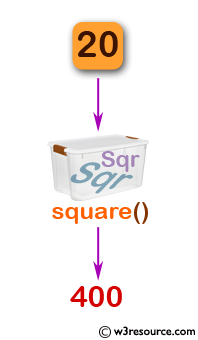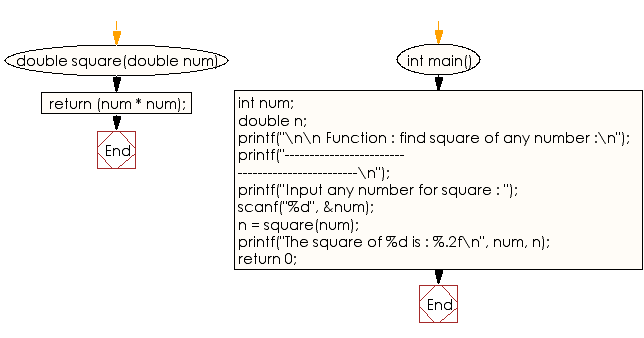﻿ C Program: Find the square of any number - w3resource

# C Exercises: Find the square of any number

## C Function : Exercise-2 with Solution

Write a program in C to find the square of any number using the function.

Pictorial Presentation:Sample Solution:

C Code:

``````#include <stdio.h>

double square(double num)
{
return (num * num);
}
int main()
{
int num;
double n;
printf("\n\n Function : find square of any number :\n");
printf("------------------------------------------------\n");

printf("Input any number for square : ");
scanf("%d", &num);
n = square(num);
printf("The square of %d is : %.2f\n", num, n);
return 0;
}
```
```

Sample Output:

``` Function : find square of any number :
------------------------------------------------
Input any number for square : 20
The square of 20 is : 400.00
```

Explanation:

```double square(double num) {
return (num * num);
}
```

The above function ‘square’ takes a single argument of type double, named num. It computes the square of the input number by multiplying num with itself and then returns the resulting value. Essentially, this function returns the square of a given number.

Time complexity and space complexity:

The time complexity of the function ‘square’ is O(1), as the computation it performs is constant and independent of the input size.

The space complexity of the function ‘square’ is also O(1), as it only uses a fixed amount of memory to store the local variable holding the computed result.

Flowchart:C Programming Code Editor:

What is the difficulty level of this exercise?

Test your Programming skills with w3resource's quiz.

﻿